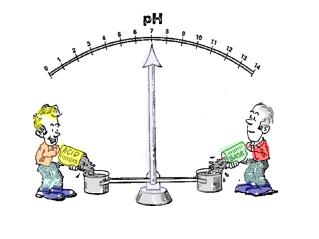Download PresentationDefinition pH and pOH. Given pH, pOH, [H 3 O + ] or [OH¯], calculate the remaining values.

Loading in 2 Seconds...

# Definition pH and pOH. Given pH, pOH, [H 3 O + ] or [OH¯], calculate the remaining values. - PowerPoint PPT Presentation

Definition pH and pOH. Given pH, pOH, [H 3 O + ] or [OH¯], calculate the remaining values. Calculate Ka/Kb, given the pH or pOH and the concentration of a weak acid solution. Describe how an acid-base indicator works in terms of the colour shifts and Le Chatelier's Principle.I am the owner, or an agent authorized to act on behalf of the owner, of the copyrighted work described.
Download Presentation## Definition pH and pOH. Given pH, pOH, [H 3 O + ] or [OH¯], calculate the remaining values.

An Image/Link below is provided (as is) to download presentation

Download Policy: Content on the Website is provided to you AS IS for your information and personal use and may not be sold / licensed / shared on other websites without getting consent from its author.While downloading, if for some reason you are not able to download a presentation, the publisher may have deleted the file from their server.

- - - - - - - - - - - - - - - - - - - - - - - - - - E N D - - - - - - - - - - - - - - - - - - - - - - - - - -
Presentation Transcript

1. Definition pH and pOH. • Given pH, pOH, [H3O+] or [OH¯], calculate the remaining values. • Calculate Ka/Kb, given the pH or pOH and the concentration of a weak acid solution. • Describe how an acid-base indicator works in terms of the colour shifts and Le Chatelier's Principle.

2. KW = [H+][OH¯] KW = 1.0 x 10-14 Acids / bases dissolve in water - increase [H+] / [OH-] and cause an equilibrium shift. Acidic - [H+] is greater than the [OH-] Basic - [OH-] is greater than [H+] H2O(l) H+(aq) + OH¯(aq)

3. Acidic - [H3O+] > [OH¯] Alkaline (basic) - [OH¯] > [H3O+] Neutral - [OH¯] = [H3O+] 1909 - Soren Sorensen developed a simplified system for the degree of acidity of a solution. pH - the potenz (power) of hydrogen - German potentia hydrogenii - Latin

4. Convenient way to express [H+] pH = -log [H+] Similarly, the concentration of hydroxide can be expressed as pOH: pOH = -log [OH-] pOH has its own scale – like pH – that runs from 0-14 for strength of bases.

5. [H+][OH-] = 1.0 x 10-14 pH + pOH = 14 Special case - pH can be read straight from the value of the [H+]. [H+] = 1.0 x 10-x then pH = x [OH-] = 1.0 x 10-x then pOH = x

6. Remember, in a neutral solution pH & pOH = 7 Acidic - pH < 7 Basic - pH > 7

7. Calculate the pH of an HCl solution whose concentration is 5.0 x 10-6 mol/L. remember strong acids are 100% dissociating: [HA]i = [H+]e = [A-]e pH = -log[H+] = -log(5.0 x 10-6 M) = -(-5.30) = 5.30

8. The pH of a solution is 3.25. Calculate the hydrogen ion concentration in the solution. [H3O+] = 10-pH Find the “10 x” button on your calculator. = 10-3.25 = 5.6 x 10-4 M ** also means: [OH-] = 10-pOH

9. The pH of a solution is 10.30, what is the hydroxide ion concentration? OR solved this way pOH = 14.00 - pH pOH = 14.00 - 10.30 = 3.70 [H+] = 10-pH [OH-] = KW [H+] [OH-] = 10-pOH = 10-3.70 = 2.0 x 10-4 mol/L

10. Mg(OH)2 (s) Mg2+(aq) + 2 OH-(aq) xi xf 2xf What is the pH of 5.0 x 10-5 M Mg(OH)2 solution? remember strong bases are 100% dissociating [OH-] = 2x = 2(5.0 x 10-5 M) = 1.0 x 10-4 M pOH = -log[OH-] = 4.00 pH = 14.00 - 4.00 = 10.00

11. YOUR ACID/BASE FORMULAE: • Kw = [H+][OH-] = 1.0 x 10-14 • The pH and pOH scales goes from 0 - 14. • pH = -log[H+] and[H+] = 10-pH • pOH = -log[OH-] and[OH-] = 10-pOH • pOH + pH =14

12. Measuring pH There are two ways to measure pH: pH Meters Indicators The [H+] inside the probe (reference electrode) is compared to [H+] outside the probe. Probe must be calibrated first. (inserted into known pH solution)

13. An indicator is a weak acid or base that undergoes a colour change when they gain or lose hydrogen ions. Natural pH indicators Beets, Blackcurrant juice, Blueberries , Carrots , Cherries , Curry Powder , Delphinium Petals , Geranium Petals , Grapes , Horse Chestnut Leaves , Hydrangea , Morning Glories , Onion , Pansy Petals , Petunia Petals , Poison Primrose , Poppy Petals , Purple Peonies , Rayhan Leaves , Red cabbage , Red Radish , Rhubarb , Rose Petals , Strawberries ,Tea , Thyme , Turmeric , Tulip Petals , Violet Petals

14. Red Litmus stays red in the presense of an acid.

15. It turns blue in presense of a base.

16. Phenolphthalein is a commonly used indicator. Weak acid is colourless and its conjugate base ion is bright pink.

17. Kw = [H+][OH-] = 1.0 x 10-14 • pH = -log[H+] and[H+] = 10-pH • pOH = -log[OH-] and[OH-] = 10-pOH • pOH + pH =14 • Indicators are weak acids or bases that change • colour in response to changing hydronium ion • concentrations.